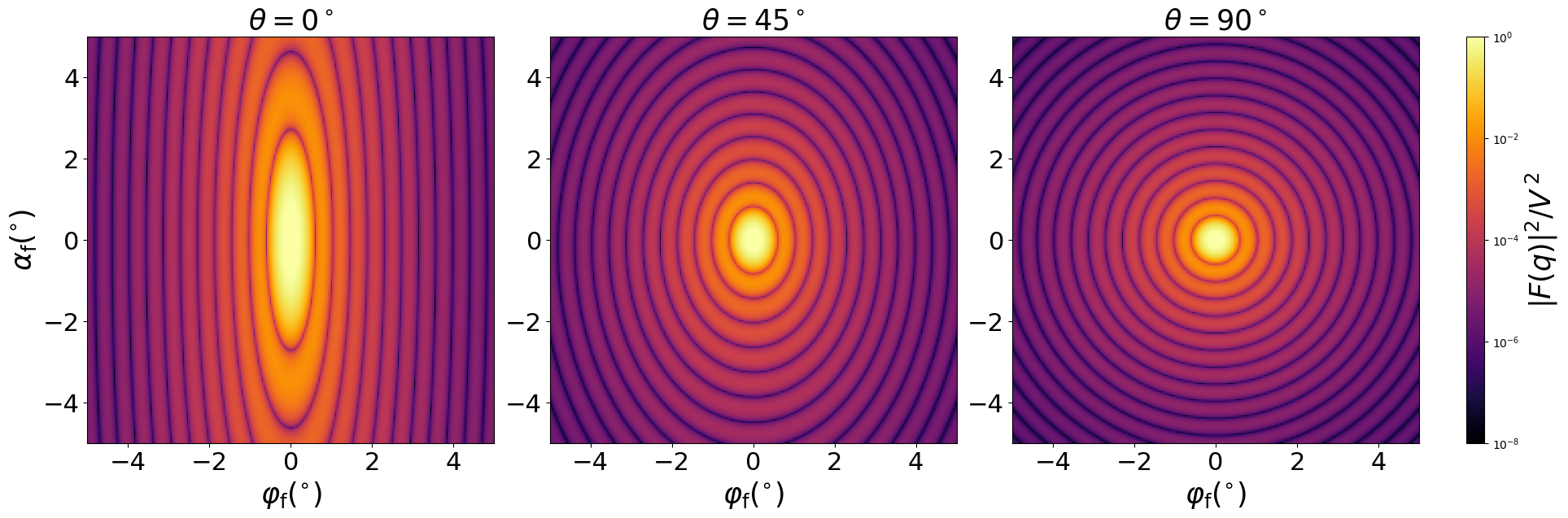### Spheroid

A full spheroid, generated by rotating an ellipse around the vertical axis.#### Constructor

Spheroid(R, H)


Parameters:

• H, height

#### Usage

As for any other Form factor.

#### Implementation

Class Spheroid inherits from the interface class IFormFactor .

Form factor is computed as

$$F(\mathbf{q})=4\pi R^2h \exp(iq_zh) \dfrac{\sin(s) - s \cos(s)}{s^3} ,$$ with the notation $$h:=H/2, \quad s := \sqrt{(Rq_x)^2 + (Rq_y)^2+ (hq_z)^2}.$$

Volume has been validated against $$V=\dfrac{2\pi}{3} R^2H.$$

More special:

More general:

#### Example

Scattering by uncorrelated, oriented spheroids for horizontal incidence. Rotation around $y$ axis:Generated by Examples/ff/Spheroid.py .

#### History

Replicates the “Full spheroid” in IsGISAXS [manual, Eq. 2.36; Renaud 2009, Eq. 227], except for wrong factors of 2 in their volume formula and form factor implementation, and “Spheroid” in FitGISAXS [Babonneau 2013]. Up to BornAgain 1.16, our form factor computation followed IsGISAXS in using numeric integration in the $z$ coordinate. In BornAgain, named “FullSpheroid” up to version 1.19.